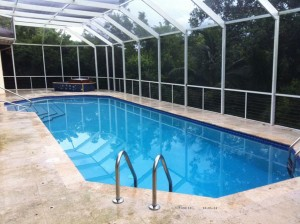# What Is The Square Footage Of My Inground Swimming Pool

Written on:April 7, 2022

### What is the Square Footage of my Inground Swimming Pool?Knowing the Square Footage of a swimming pool is very important when you need to paint it.

Estimating the amount of Paint your project will require depends on the smoothness of the surface, the number of coats recommended for a particular coating and the total square feet of the area to be painted.

Refer to the specific paint product you plan to use for details on whether a primer coat is needed, coverage per gallon and recommended number of coats. Use the information below for an estimate of the total square feet of your swimming pool’s interior.

This formula is approximate since pools most likely have many round corners and steps, but should work for determining the amount paint or tiles needed to finish a pool. We recommend rounding up on the measurements and we always recommend getting an extra gallon of paint just in case. You don’t want to run out of paint in the middle of your project.

The formula is as follows:

(L x W) + (L x Avg Depth x 2) + (W x shallow depth) + (W x deep depth) = Total square footage of surface area of all the pool sides and bottom.

To determine average depth in a pool where the bottom slopes: Measure the shallow end and measure the deep end. Add them together and divide by 2. This is the average depth of your pool.

Below is an example using a 16 x 32 pool with a Shallow depth of 4′, a deep depth of 8′ and an average depth of 6′ feet

L x W = (32 x 16) = 512

L x Avg. Depth x 2 = (32 x 6 x 2) = 384

W x Shallow Depth = (16 x 4) = 64

W x Deep Depth = (16 x 8) = 128

512 sqft for the bottom + 384 sqft (the two long sides) + 64 sqft (small side) + 128 sqft (deep side) = 1088 SQFT.

If you have any questions please feel free to give us a call at 1-800-876-7647